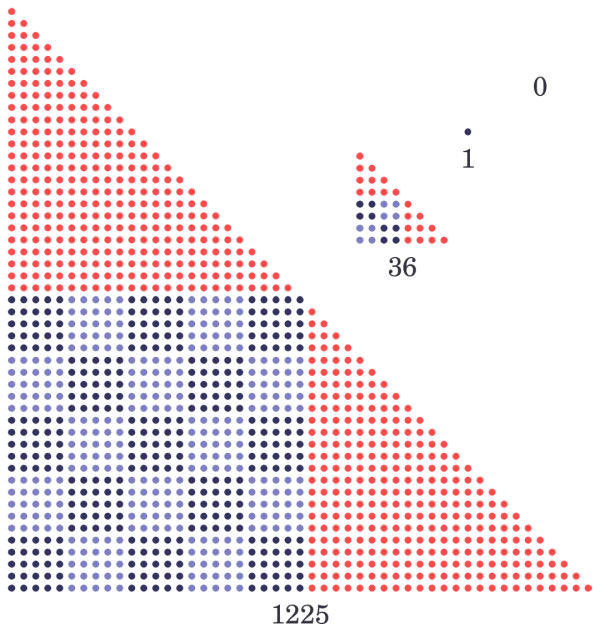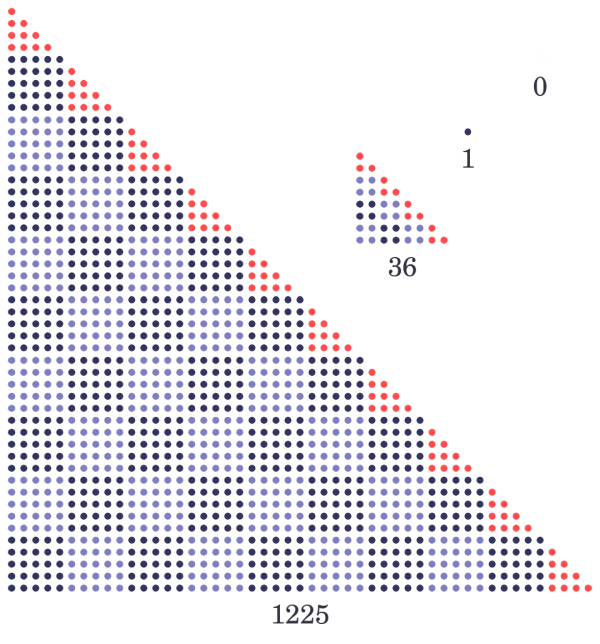# Dani’s OEIS adventures: triangular square numbers

Hi! I’m Dani Poveda. This is my first post here on The Aperiodical. I’m from Spain, and I’m not a mathematician (I’d love to be one, though). I’m currently studying a Spanish equivalent to HNC in Computer Networking. I’d like to share with you some of my inquiries about some numbers. In this case, about triangular square numbers.

I’ll start at the beginning.

I’ve always loved maths, but I wasn’t aware of the number of YouTube maths channels there were. During the months of February and March 2016, I started following some of them (Brady Haran’s Numberphile, James Grime and Matt Parker among others). On July 13th, Matt published the shortest maths video he has ever made:

Maybe it’s a short video, but it got me truly mired in those numbers, as I’ve loved them since I read The Number Devil when I was 8. I only needed some pens, some paper, my calculator (Casio fx-570ES) and if I needed extra help, my laptop to write some code. And I had that quite near me, as I had just got home from tutoring high school students in maths.

I’ll start explaining now how I focused on this puzzle trying to figure out a solution.

The first thing that I thought of was to take my calculator and enter $\sqrt{\frac{x(x+1)}{2}}$, letting $x$ be an integer greater than $8$, as $\sqrt{\frac{8(8+1)}{2}}=6$ (and that’s the one Matt used to introduce the challenge). That way I found the next one, $1225$, for $x=49$. After trying with some more values for $x$, I got tired and I decided to write it in PHP. In this way I found the first few terms of the sequence: $0$, $1$, $36$, $1225$, $41616$, $1413721$, $48024900$, $1631432881$…

I went on naming, square numbers as $S$, triangular numbers as $T$ and square triangular numbers as $ST$. I then thought of addressing this problem, $ST_{n}=S_{i}=T_{j}$, by expressing $S$ and $T$ as series:

$S_{i}=i^2=\sum_{x=0}^{i-1} 2x+1$ $T_{j}=\frac{j \cdot (j+1)}{2}=\sum_{x=0}^{j} x$

As each square number is a sum of consecutive odd numbers and each triangular number is a sum of consecutive numbers, I focused on the different elements in each sum, noticing that parity of triangular numbers is important and that there has to be a sum of consecutive odd numbers that equals a sum of consecutive even numbers. For example:

$ST_{2}=36=S_{6}=1+3+5+7+\underline{9}+\underline{11}$

$ST_{2}=36=T_{8}=1+\underline{2}+3+\underline{4}+5+\underline{6}+7+\underline{8}$

Underlined numbers are all different summands between both sums, so they add to the same result: $9+11=2+4+6+8=20$. Considering the sum of consecutive even numbers, we always obtain twice a triangle, $2+4+6+8=2 \cdot (1+2+3+4)=2 \cdot T_{4}$. Knowing that and doing the same thing for $1225$, $41616$, $\ldots$ I looked for a pattern in the series and I found that for every $ST_n = S_{i} = T_{j}$:

$\sum_{x={\frac{j+(j \bmod 2)}{2}}}^{i-1} 2x+1 = \sum_{x=0}^{{\frac{j-(j \bmod 2)}{2}}} 2x = 2 \cdot T_{\frac{j-(j \bmod 2)}{2}}$

For $n \gt 1$, I noticed that $\frac{j+(j \bmod 2)}{2}$ is a square number and it divides $ST_{n}$, resulting in another square number that equals $j+((j+1) \bmod 2)$, so every square triangular number is a product of two square numbers:

\begin{align} ST_{2} &= 36 = S_6 = T_8 \\ &= \frac{8+0}{2} \cdot (8+1) = 2^2 \cdot 3^2 \end{align} \\ \sum_{x=\frac{8+0}{2}}^{6-1} \left( 2x+1 \right) = \sum_{x=0}^{ \frac{8-0}{2}} \left( 2x \right) = 2 \cdot T_{4} = 20
\begin{align} ST_3 &= 1225 = S_{35} = T_{49} \\ &= \frac{49+1}{2} \cdot (49+0) = 5^2 \cdot 7^2 \end{align} \\ \sum_{x=\frac{49+1}{2}}^{35-1} \left( 2x+1 \right) = \sum_{x=0}^{\frac{49-1}{2}} \left( 2x \right) = 2 \cdot T_{24} = 600
\begin{align} ST_4 &= 41616 = S_{204} = T_{288} \\ &= \frac{288+0}{2} \cdot \left( 288+1 \right) = 12^2 \cdot 17^2 \end{align} \\ \sum_{x=\frac{288+0}{2}}^{204-1} \left( 2x+1 \right) = \sum_{x=0}^{\frac{288-0}{2}} \left( 2x \right) = 2 \cdot T_{144} = 20880
\begin{align} ST_5 &= 1413721 = S_{1189} = T_{1681} \\ &= \frac{1681+1}{2} \cdot (1681+0) = 29^2 \cdot 41^2 \end{align} \\ \sum_{x=\frac{1681+1}{2}}^{1189-1} \left( 2x+1 \right) = \sum_{x=0}^{\frac{1681-1}{2}} \left( 2x \right) = 2 \cdot T_{840} = 706440

At this point I was freaking out, because I was feeling I was so close to a formula for these numbers – I was getting something quite amazing but I couldn’t be sure because my method was so chaotic. Then I tried to find a general term for a sequence $a$ which has those factors in increasing order, that is $0$, $1$, $1$, $2$, $3$, $5$, $7$, $12$, $17$, $29$, $41$, $70$, $99$, $169$, $239$…

I simply defined a sequence $a$ considering $a_{0}=0$, $a_{1}=1$ and $a_{2}=1$ because I noticed how the numbers were growing and I thought it might be similar to Fibonacci numbers, but I had no idea what sequence this was. It was quite difficult to get a general formula for the terms in this sequence, but after a while, I finally managed to find it. Having $n > 2$:

$a_{n}=a_{n-1-((n-1) \bmod 2)}+2^{(n-1) \bmod 2} \cdot a_{n-2-((n-1) \bmod 2)}$

Nice fact: $a_{13} = 13^2$. I guess this only happens for $n=13$, but I’m not sure.

Finally I was able to write $i$ and $j$ in $ST_{n}=S_{i}=T_{j}$ for a given $n>0$, considering $ST_{0}=0$:

$i=a_{2n-1} \cdot a_{2n} \hspace{5pt} ; \hspace{4pt} n > 0$ $j=2 \cdot (a_{2n-1})^2 – ({a_{2n-1}} \bmod 2) \hspace{5pt} ; \hspace{4pt} n > 0$

I had finally solved it! I thought I should review everything to check I had everything right. After re-reading it several times, it seemed everything was alright. While I was looking again at the equivalent sum of consecutive odd numbers and sum of consecutive even numbers, I noticed this was twice a triangle. That fact got my attention, and I thought: ‘what if I subtract those two triangles from each corresponding square triangular number?’ I imagined it graphically, with each $ST_{n}$ for $n>2$ and I obtained these results: $16$, $625$, $20736$, $707281$, $24010000$, $815730721$, $\ldots$

I recognised the first two results – I knew they were $2^4$ and $5^4$, but I couldn’t imagine they were all going to be fourth powers: $2^4$, $5^4$, $12^4$, $29^4$, $70^4$, $169^4$…

‘Wow, this is truly nice,’ I thought. After discovering this pattern I checked for $ST_{1}$ and it worked too as it’s trivial, so I realised I had conjectured by chance some more formulae based on the sequence $a$:

$ST_{n}=(a_{2n-1})^4+\sum_{x=(a_{2n-1})^2}^{(a_{2n-1} \cdot a_{2n})-1} \left( 2x+1 \right) \hspace{5pt} ; \hspace{4pt} n > 0$
$ST_{n}=(a_{2n-1})^4+\sum_{x=0}^{(a_{2n-1})^2 – (a_{2n-1} \bmod 2)} \left( 2x \right) \hspace{5pt} ; \hspace{4pt} n > 0$
$ST_{n}=(a_{2n-1})^4+2 \cdot T_{(a_{2n-1})^2 – (a_{2n-1} \bmod 2)} \hspace{5pt} ; \hspace{4pt} n > 0$

I noticed then that I only needed the terms of the sequence $a$ in odd positions to write the last two formulae, but I didn’t (and don’t) know how to prove everything by induction.

So I drew the two first square triangular numbers as rectangular triangular shapes, and I discovered that each fourth power is graphically a square of squares with two identical triangles attached to two of its sides in such a way that the square’s corner between them either is or isn’t part of the whole shape’s hypotenuse, depending on the parity of the total number of elements.Having spent the most part of two days working on this, I searched on the internet for everything I could find about these numbers. I was so surprised when I couldn’t find anything related to these patterns, except for one of the formulas that I found, $ST_{n}=(a_{2n-1} \cdot a_{2n})^2 \hspace{3pt} ; \hspace{2pt} n > 0$, and it was a contribution by Hugh Darwen, approved in the OEIS on February 23, 2012. But the rest of the work I’d done… I couldn’t find anything like it. I looked for papers that may have something related to this, but again… nothing. Maybe I should have looked harder, because I couldn’t believe I’d actually been able to notice something that nobody has noticed before… I found out that sequence $a$ is almost A002965, but not exactly, as one initial term is missing. And I discovered too that $a$ terms in odd positions correspond to Pell numbers, which I didn’t know before… I found out about the Silver ratio and other stuff that I find amazing! I’m glad that I saw Matt’s video in the first place.

Then I decided to sign up for an account on the OEIS to publish these formulae in a draft, and to write about this in my OEIS profile page. When I received an email from the OEIS telling me that Neil Sloane had approved my changes to sequence A001110… I was shocked. Really. I wasn’t expecting anything like that. I find all of the work I’ve done to be kind of basic, because I took as a starting point something I learned in a book I read when I was 8… Is this possible? It seems to be.

After that contribution, I wanted to generalise the formula $ST_{n}=(a_{2n-1})^4+2 \cdot T_{(a_{2n-1})^2 – (a_{2n-1} \bmod 2)} \hspace{5pt} ; \hspace{4pt} n > 0$, and since that moment I’ve been contributing more sequences and formulae. In fact, I first generalised this formula in sequence A275496 for every fourth power (every square of squares). I also tessellated each whole triangle and I found another formula for square triangular numbers. Letting $P_{n}$ be the $n$th Pell number:

$ST_{n}={{P_{n}}^2 \cdot T_{2 \cdot P_{n} – 1} + 2 \cdot P_{n} \cdot T_{P_{n} – (n \bmod 2)}} \hspace{5pt} ; \hspace{4pt} n > 0$After that, I generalized all these inquiries publishing sequence A276914, also making an illustration for the OEIS where I explain the main pattern.

I hope Matt sees all this (he already knows a bit about this, as I’ve written to him by email). I’ve been making more inquiries about other numbers, such as centered polygonal numbers (A276916 are centered square numbers based in A276914, by the way). I’ve posted some images on Twitter about really really nice patterns related to prime numbers and stuff.

Maybe I can write another post in future about some of my number games!

We showed Dani’s article to Matt Parker. He says:Congratulations to Dani for such a fantastic explanation of the mathematics he found in triangle-square numbers! It was nice to see how he started the same way I did when I first investigated these numbers but then very quickly explored new directions I’d never considered.
I love it when people play around with mathematics because of something they have seen online (or noticed in normal life), but it is always extra-amazing if they then take the time to share their experiences with other people. So thanks again to Dani for emailing me about what he was doing, and writing it up for The Aperiodical.

•#### Dani Poveda

Checking what everyone takes for granted. Here goes one of my favourite quotes: “A man with a new idea is a crank until the idea succeeds” (Mark Twain). By the way, I love maths.

### 2 Responses to “Dani’s OEIS adventures: triangular square numbers”

1.Anonymous

The number1